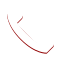Data Science & data analytics for beginners 2020-04-15T06:28:06+00:00

# Data Science for beginners

Get familiar with Data Science & data analytics learning Techniques for beginners.

INR 40,000 + Taxes

## Why pursue Data Science:

Data Science is the most preferred subject in the industry as of trend having a huge demand for professionals who got trained in it.

There are so many data science algorithms to build predictive models, such as logistic regression, linear regression, decision trees and random forests. The potential of data science is limitless – spanning across industries, having various roles and functions to perform.

# Best Data Science Training Institute in Hyderabad

### What is Data Science? Why is it important?

Data science is a deep study of the massive amount of data, which includes extracting meaningful insights from raw, structured and unstructured data, organized and unstructured information that is prepared to utilize the logical strategy. It is a multidisciplinary field that uses tools and techniques to control the information with the goal that you can discover something new and meaningful. Data science utilizes the most powerful hardware, programming systems and most proficient algorithms to solve the data related issues.

An ever-increasing number of organizations are coming to understand the significance of data science, AI and Machine learning. Regardless of industry or size, organizations that desire to stay focused in the time of enormous information need to efficiently develop and implement data science capabilities or risk being left behind.

Why learn Data Science at Integrum Litera!

Integrum litera is a leading data science training institute in Hyderabad covering the total Data Science lifecycle concepts from Data Collection, Data Extraction, Data Cleansing, Data Exploration, Data Transformation, Feature Engineering, Data Integration, Data Mining, building prediction models, Data Visualization and deploying. Our data science training programme has all the fundamental course details such as simple Linear regression, Multiple linear regression, Logistic regression, Basic statistics, Decision tree, Case studies, Algorithms on classification, time series, multi-class prediction algorithm, Neural networks, Vector machines and more. Data science coaching at Integrum would enhance the technical skills on Artificial Intelligence, Git, Python, Machine Learning, Deep Learning, Tableau, R, SAS, Excel, and Unix. Data science online training at Integrum litera is an impressing factor for all the newcomers and our training materials are more helpful to the students who are looking for certification in data science.

## Course Details

#### Data Science course allows you to learn

• Understand what data science is and diverse applications of it.
• Common data science terminologies or syntax.

#### Python for data science – popular data science programming language:

• Python from scratch – programming experience not required!
• Understand how to use Jupyter Notebooks for data science and also get familiar with Python libraries like Numpy, Pandas

#### Core Statistics for data science such as Descriptive Statistics and Inferential Statistics

• Measures of central tendency such as mean, median, mode
• Standard deviation
• Outlier detection
• Correlation
• Hypothesis Testing
• Confidence Intervals & margin of error
• Chi-squared test

#### Probability concepts for Data Science

• Introduction to Probability and its concepts for data science
• Central Limit Theorem
• Bernoulli Trials

#### Basics to core machine learning algorithms for data science

• Basics of machine learning model building and Types of predictive models in data science
• Overview of end-to-end data science process
• Data extraction and data cleaning
• Linear regression and Logistic regression
• Decision trees
• Random forests

#### What is Data Science

Data Science is a combination of algorithms, tools and machine learning techniques which helps you to discover common basic patterns from the given raw data.

#### What is the Decision Tree algorithm?

A decision tree is a popular supervised machine learning algorithm. It is mainly utilized for Regression and Classification.

#### What is logistic regression in Data Science?

Logistic regression is a statistical analysis method used to anticipate a data value based on prior perceptions of a data set.Call Now